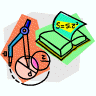## Mathematics, Department of## Department of Mathematics: Faculty Publications

### Algebraic Isomorphisms and Spectra of Triangular Limit Algebras

2001

#### Citation

Indiana University Mathematics Journal c , Vol. 50, No. 3 (2001)

2001 MATHEMATICS SUBJECT CLASSIFICATION: Primary: 47L40; secondary: 47L50.

#### Abstract

We show that the spectrum of a triangular regular limit algebra (TAF algebra) is an invariant for algebraic isomorphism. Combining this with previous results provides a striking rigidity property: two triangular regular limit algebras are algebraically isomorphic if and only if they are isometrically isomorphic. A consequence of spectral invariance is a structure theorem for isomorphisms between limit algebras.

The proof of the main theorem makes use of a characterization of the completely meet irreducible ideals of a TAF algebra and a dual space formulation of the spectrum.

COinS